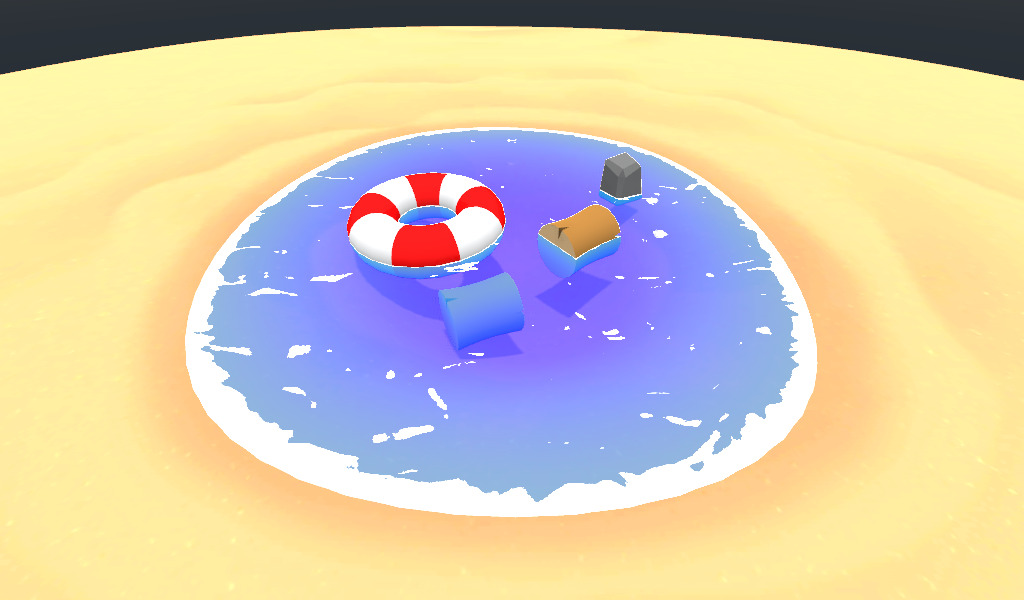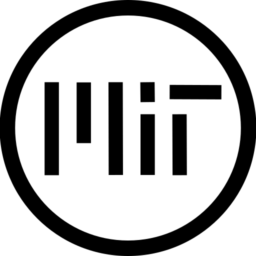This is a port from the original unity shader by Erik Roystan Ross explained in this article, and hosted on github with Unlicense License

There are still some issues:

• Godot 3 doesn’t has NORMAL texture from the Camera on Spatial shaders, so the normal calculations might be not correct

I encourage all people to contribute to this and make it better , code and project is hosted on github

``````/**
* Ported from the original unity shader by Erik Roystan Ross
* https://roystan.net/articles/toon-water.html
* Camera Depth taken from Bastiaan Olij's video on: https://www.youtube.com/watch?v=Jq3he9Lbj7M
*/

const float SMOOTHSTEP_AA = 0.01;

uniform sampler2D surfaceNoise;
uniform sampler2D distortNoise;

uniform float beer_factor = 0.8;

uniform float foam_distance = 0.01;
uniform float foam_max_distance = 0.4;
uniform float foam_min_distance = 0.04;
uniform vec4 foam_color: hint_color  = vec4(1.0);

uniform vec2 surface_noise_tiling = vec2(1.0, 4.0);
uniform vec3 surface_noise_scroll = vec3(0.03, 0.03, 0.0);
uniform float surface_noise_cutoff: hint_range(0, 1) = 0.777;
uniform float surface_distortion_amount: hint_range(0, 1) = 0.27;

uniform vec4 _DepthGradientShallow: hint_color = vec4(0.325, 0.807, 0.971, 0.725);
uniform vec4 _DepthGradientDeep: hint_color = vec4(0.086, 0.407, 1, 0.749);
uniform float _DepthMaxDistance: hint_range(0, 1) = 1.0;
uniform float _DepthFactor = 1.0;

varying vec2 noiseUV;
varying vec2 distortUV;
varying vec3 viewNormal;

vec4 alphaBlend(vec4 top, vec4 bottom)
{
vec3 color = (top.rgb * top.a) + (bottom.rgb * (1.0 - top.a));
float alpha = top.a + bottom.a * (1.0 - top.a);

return vec4(color, alpha);
}

void vertex() {
viewNormal = (MODELVIEW_MATRIX * vec4(NORMAL, 0.0)).xyz;
noiseUV = UV * surface_noise_tiling;
distortUV = UV;
}

void fragment(){
float depth = texture(DEPTH_TEXTURE, SCREEN_UV).r;
depth = depth * 2.0 - 1.0;
depth = PROJECTION_MATRIX / (depth + PROJECTION_MATRIX);
depth = depth + VERTEX.z;
depth = exp(-depth * beer_factor);
depth = 1.0 - depth;

// Still unsure how to get properly the NORMAL from the camera
// this was my best attempt
vec3 existingNormal = vec3(dFdx(depth), dFdy(depth), 0);

float normalDot = clamp(dot(existingNormal.xyz, viewNormal), 0.0, 1.0);
float foamDistance = mix(foam_max_distance, foam_min_distance, normalDot);

float foamDepth = clamp(depth / foamDistance, 0.0, 1.0);
float surfaceNoiseCutoff = foamDepth * surface_noise_cutoff;

vec4 distortNoiseSample = texture(distortNoise, distortUV);
vec2 distortAmount = (distortNoiseSample.xy * 2.0 -1.0) * surface_distortion_amount;

vec2 noise_uv = vec2(
(noiseUV.x + TIME * surface_noise_scroll.x) + distortAmount.x ,
(noiseUV.y + TIME * surface_noise_scroll.y + distortAmount.y)
);
float surfaceNoiseSample = texture(surfaceNoise, noise_uv).r;
float surfaceNoiseAmount = smoothstep(surfaceNoiseCutoff - SMOOTHSTEP_AA, surfaceNoiseCutoff + SMOOTHSTEP_AA, surfaceNoiseSample);

float waterDepth = clamp(depth / _DepthMaxDistance, 0.0, 1.0) * _DepthFactor;

vec4 surfaceNoiseColor = foam_color;
surfaceNoiseColor.a *= surfaceNoiseAmount;
vec4 color = alphaBlend(surfaceNoiseColor, waterColor);

ALBEDO = color.rgb;
ALPHA = color.a;
}``````
###### Tags
toon, waterThe shader code and all code snippets in this post are under MIT license and can be used freely. Images and videos, and assets depicted in those, do not fall under this license. For more info, see our License terms.

### fBm Toon WaterInline FeedbacksNekotoArts
1 year ago

I actually can’t believe it! I saw this on GitHub a few days ago and was really curious if it could be recreated in Godot, AND THEN I SEE THIS TODAY! Absolutely beautiful!11 months ago

I tried to do the exact same thing as you and failed. So grateful that you shared this!!!DeveloperOats
2 months ago

Awesome Shader. Just found it and added to my game. It looks sooo nice 🙂
Thanks for the work and the code.

How do I share a screenshot ?Crabsatos
5 days ago

If you are wondering how to make this shader work in Godot 4 (alpha), you need to make the following changes:

• replace “hint_color” with “source_color”
• remove this line: depth = depth * 2.0 – 1.0;
• (Vulkan depth texture has range 0 to 1 already)